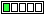All about flooble | fun stuff | Get a free chatterbox | Free JavaScript | Avatarsperplexus dot infoplay with digit (Posted on 2013-09-10)Let A be a 2n-digit number whose digits are all 1's and let B be a n-digit number whose digits are all 2's.Show that A-B is a perfect square.

 No Solution Yet Submitted by Danish Ahmed Khan No RatingComments: ( Back to comment list | You must be logged in to post comments.)solution Comment 1 of 1

A = sum(10^k,k=0 to 2n-1)
A=(10^(2n)-1)/9

B=2*sum(10^k,k=0 to n-1)
B=2*(10^n-1)/9

A-B=(10^(2n)-1)/9 - 2*(10^n-1)/9
A-B=(10^(2n)-2*10^n+1)/9
A-B=(10^n-1)^2/3^2
A-B=[(10^n-1)/3]^2
A-B=[3*(10^n-1)/9]^2
A-B=[3*sum(10^k,k=0 to n-1)]^2
A-B=[333....3]^2
thus the square root of A-B is the number consisting of n 3's.  Thus A-B is a perfect square

 Posted by Daniel on 2013-09-10 10:16:02Please log in:

 Search: Search body:
Forums (0)